# High School Math : High School Math

## Example Questions

### Example Question #14 : Integers And Types Of Numbers

Which of the following is not an integer?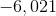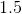Explanation:

Integers are all counting numbers, and include bothand all negative counting numbers. Thus, keeping this definition in mind, we can see that the value which is not an integer issince it has digits after the decimal point.

### Example Question #61 : High School Math

What is the difference between 8 and -2?

-6

10

4

6

-10

10

Explanation:

Since we want to find the difference between 8 and -2, we want to subtract -2 from 8. Since subtracting is equivalent to adding the same number with the opposite sign, we have the below: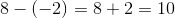### Example Question #62 : High School Math

Find the difference.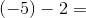Explanation:

We are subtracting here, so it is important to remember that subtracting is really just adding the same number with opposite sign. Thus we can think of the problem as the following: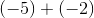, which we know is equal to.

### Example Question #63 : High School Math

What is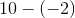?Explanation:

Recall that subtracting integers is equivalent to adding the inverse. The inverse of a negative number is the positive number with the same magnitude.

Thus, our problem is equivalent to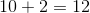.

### Example Question #64 : High School Math

Evaluate the following expression: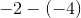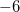Explanation:

Recall that subtracting is equivalent to adding the inverse: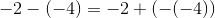The inverse of a negative number is a positive number: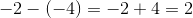### Example Question #65 : High School Math

What is: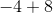Explanation:

Recall the placement of numbers on the number line.isspaces to the left of, and then we add, resulting in movement to the right.

When we add these spaces we end up goingspaces back toand thenadditional spaces to end at### Example Question #66 : High School Math

What is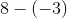?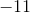Explanation:

Recall that subtracting a negative number is equivalent to adding the opposite. Thus,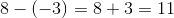### Example Question #67 : High School Math

What is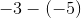Explanation:

Recall that subtracting negative numbers is equivalent to adding the opposite. Then, we have that: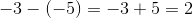### Example Question #68 : High School Math

Solve the expression below.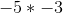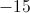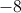Explanation:

In this case, it is key to recall the rules for multiplying or dividing with negative values.

Negative * Positive = Negative

Negative * Negative = Positive

Positive * Positive = Positive

In this case, we are multiplying two negative numbers; thus the answer should be a positive number. To find the value, we can simply multiply the terms without their negatives.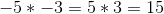### Example Question #69 : High School Math

Simplify: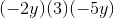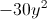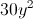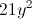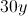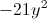Explanation:

First multiply all the numbers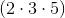.

If there is an even amount of negative signs, then the product will be positive. If there is an odd amount of negative signs, then the product will be negative.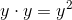Therefore, the answer is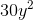.